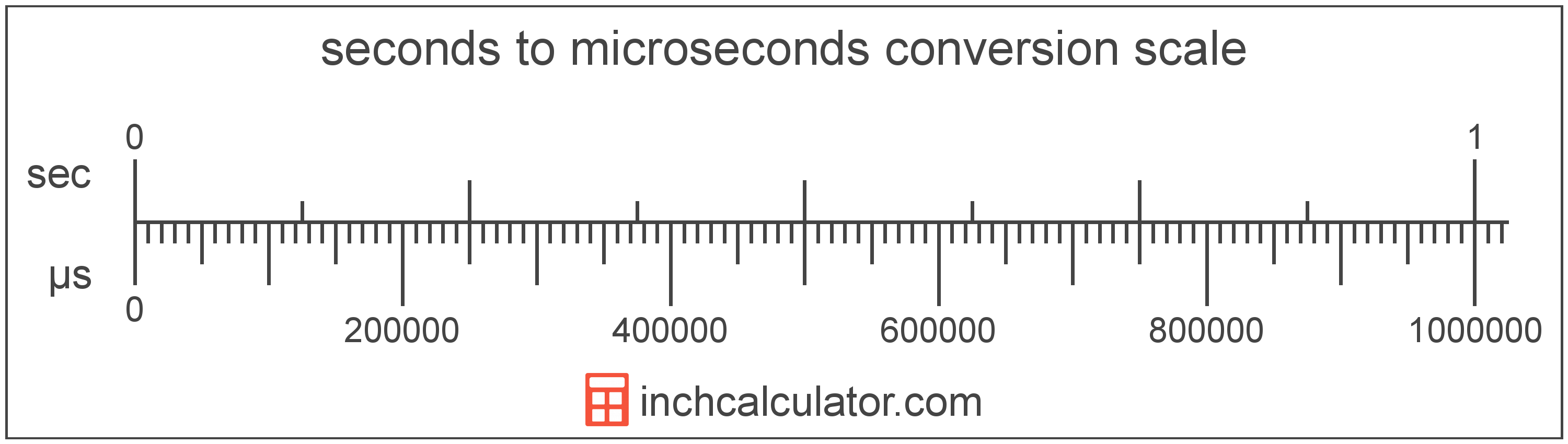# Seconds to Microseconds Converter

Enter the time in seconds below to get the value converted to microseconds.

Results in Microseconds:1 s = 1,000,000 µs

Do you want to convert microseconds to seconds?

## How to Convert Seconds to Microseconds

To convert a measurement in seconds to a measurement in microseconds, multiply the time by the following conversion ratio: 1,000,000 microseconds/second.

Since one second is equal to 1,000,000 microseconds, you can use this simple formula to convert:

microseconds = seconds × 1,000,000

The time in microseconds is equal to the time in seconds multiplied by 1,000,000.

For example, here's how to convert 5 seconds to microseconds using the formula above.
microseconds = (5 s × 1,000,000) = 5,000,000 µs### How Many Microseconds Are in a Second?

There are 1,000,000 microseconds in a second, which is why we use this value in the formula above.

1 s = 1,000,000 µs

## What Is a Second?

The second has historically been defined as 1/60 of a minute or 1/86,400 of a day. According to the SI definition, the second is the duration of 9,192,631,770 oscillations of the frequency of light emitted from the atomic transition between the two hyperfine levels of the unperturbed ground state of the caesium 133 atom.

The second is the SI base unit for time in the metric system. Seconds can be abbreviated as s, and are also sometimes abbreviated as sec. For example, 1 second can be written as 1 s or 1 sec.

## What Is a Microsecond?

One microsecond is equal to 1/1,000 of a millisecond or 1,000 nanoseconds.

The microsecond is a multiple of the second, which is the SI base unit for time. In the metric system, "micro" is the prefix for millionths, or 10-6. Microseconds can be abbreviated as µs; for example, 1 microsecond can be written as 1 µs.

## Second to Microsecond Conversion Table

Table showing various second measurements converted to microseconds.
Seconds Microseconds
0.000001 s 1 µs
0.000002 s 2 µs
0.000003 s 3 µs
0.000004 s 4 µs
0.000005 s 5 µs
0.000006 s 6 µs
0.000007 s 7 µs
0.000008 s 8 µs
0.000009 s 9 µs
0.0000001 s 0.1 µs
0.000001 s 1 µs
0.00001 s 10 µs
0.0001 s 100 µs
0.001 s 1,000 µs
0.01 s 10,000 µs
0.1 s 100,000 µs
1 s 1,000,000 µs

## References

1. International Bureau of Weights and Measures, The International System of Units, 9th Edition, 2019, https://www.bipm.org/documents/20126/41483022/SI-Brochure-9-EN.pdf